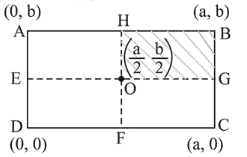# A uniform rectangular thin sheet

Question:

A uniform rectangular thin sheet $\mathrm{ABCD}$ of mass $\mathrm{M}$ has length a and breadth $b$, as shown in the figure. If the shaded portion HBGO is cut-off, the coordinates of the centre of mass of the remaining portion will be :1. (1) $\left(\frac{3 a}{4}, \frac{3 b}{4}\right)$

2. (2) $\left(\frac{5 a}{3}, \frac{5 b}{3}\right)$

3. (3) $\left(\frac{2 a}{3}, \frac{2 b}{3}\right)$

4. (4) $\left(\frac{5 a}{12}, \frac{5 b}{12}\right)$

Correct Option: , 4

Solution:

(4) With respect to point $\theta$, the $\mathrm{CM}$ of the cut-off portion

$\left(\frac{a}{4}, \frac{b}{4}\right)$

Using, $x_{\mathrm{CM}}=\frac{M X-m x}{M-m}$

$=\frac{M \times 0-\frac{M}{4} \times \frac{a}{4}}{M-\frac{M}{4}}=-\frac{a}{12}$

and $y_{C M}=-\frac{b}{12}$

So CM coordinates one

$x_{0}=\frac{a}{2}-\frac{a}{12}=\frac{5 a}{12}$'

and $y_{0}=\frac{b}{2}-\frac{b}{12}=\frac{5 b}{12}$